Example Questions

Example Question #403 : Algebra

You are told thatcan be determined from the expression: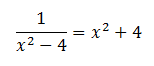Determine whether the absolute value ofis greater than or less than 2.

The quantities are equal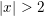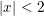The relationship cannot be determined from the information given.Explanation:

The expression is simplified as follows: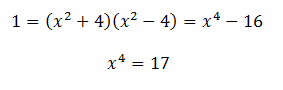Since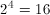the value ofmust be slightly greater for it to be 17 when raised to the 4th power.

Example Question #1 : How To Simplify An Expression

Which best describes the relationship between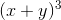and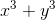if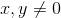?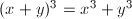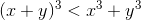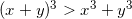The relationship cannot be determined from the information given.

The relationship cannot be determined from the information given.

Explanation:

Use substitution to determine the relationship.

For example, we could plug inand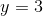.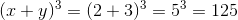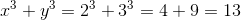So far it looks like the first expression is greater, but it's a good idea to try other values of x and y to be sure. This time, we'll try some negative values, say,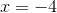and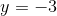.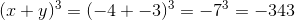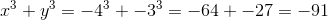This time the first quantity is smaller. Therefore the relationship cannot be determined from the information given.

Example Question #401 : Gre Quantitative Reasoning

If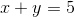and, then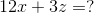Cannot be determinedExplanation:

We have three variables and only two equations, so we will not be able to solve for each independent variable. We need to think of another solution.

Notice what happens if we line up the two equations and add them together.

(x + y) + (3x – y + z) = 4x + z

and 5 + 3 = 8

Lets take this equation and multiply the whole thing by 3:

3(4x + z = 8)

Thus, 12x + 3z = 24.

Example Question #5 : How To Simplify Expressions

Simplify the following expression: x3 - 4(x2 + 3) + 15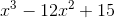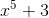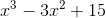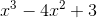Explanation:

To simplify this expression, you must combine like terms. You should first use the distributive property and multiply -4 by x2 and -4 by 3.

x3 - 4x2 -12 + 15

You can then add -12 and 15, which equals 3.

You now have x3 - 4x2 + 3 and are finished. Just a reminder that x3 and 4x2 are not like terms as the x’s have different exponents.

Example Question #25 : Simplifying Expressions

Which of the following is equivalent to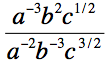?

ab/c

a2/(b5c)

b5/(ac)

abc

ab5c

b5/(ac)

Explanation:

First, we can use the property of exponents that xy/xz = xy–z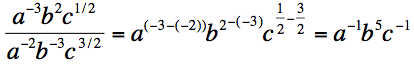Then we can use the property of exponents that states x–y = 1/xy

a–1b5c–1 = b5/ac

Example Question #26 : Simplifying Expressions

Simplify the following expression:

2x(x2 + 4ax – 3a2) – 4a2(4x + 3a)

–12a3 – 14a2x + 2x3

12a– 16a2x + 8ax2 + 2x3

–12a– 14ax2 + 2x3

–12a– 22a2x + 8ax2 + 2x3

12a– 22a2x + 8ax2 + 2x3

–12a– 22a2x + 8ax2 + 2x3

Explanation:

Begin by distributing each part:

2x(x2 + 4ax – 3a2) = 2x * x2 + 2x * 4ax – 2x * 3a2 = 2x3 + 8ax2 – 6a2x

The second:

–4a2(4x + 3a) = –16a2x – 12a3

Now, combine these:

2x3 + 8ax2 – 6a2x – 16a2x – 12a3

The only common terms are those with a2x; therefore, this reduces to

2x3 + 8ax2 – 22a2x – 12a3

This is the same as the given answer:

–12a– 22a2x + 8ax2 + 2x3

Example Question #27 : Simplifying Expressions

Simplify the following expression:

(xy)2 – x((4x)(y)– (4x)2) – 42x2

–3xy2 – 4x– 16x2

–3x2y– 16x3 – 16x2

5x2y2

–3xy– 4x3

3x2y+ 16x3 – 16x2

–3x2y– 16x3 – 16x2

Explanation:

To simplify this, we will want to use the correct order of operations. The mnemonic device PEMDAS is usually very helpful.

Parenthesis (1st)

Exponents (2nd)

Multiply, Divide (3rd)

PEMDAS tells us to evaluate parentheses first, then exponents. After exponents, we evaluate multiplication and division from left to right, and then we evaluate addition and subtraction from left to right.

Let's look at (xy)2 – x((4x)(y)– (4x)2) – 42x2

We want to start with parentheses first. We will simplify the (xy)2 by using the general rule of exponents, which states that (ab)c = acbc. Thus we can replace (xy)2 with x2y2.

x2y2 – x((4x)(y)– (4x)2) – 42x2

When we have parentheses within parentheses, we want to move from the innermost parentheses to the outermost. This means we will want to simplify the expression (4x)(y)– (4x)2 first, which becomes 4xy2 – 16x2. We can now replace (4x)(y)– (4x)2 with 4xy2 – 16x2 .

x2y2 – x(4xy2 – 16x2) – 42x2

In order to remove the last set of parentheses, we will need to distribute the x to  4xy2 – 16x2 . We will also make use of the property of exponents which states that abac = ab+c.

x2y2 – x(4xy2) – x(16x2 ) – 42x2
= x2y2 – 4x2y– 16x– 42x2

We now have the parentheses out of the way. We must now move on to the exponents. Really, the only exponent we need to simplify is –42, which is equal to –16. Remember that –42 = –(42), which is not the same as (–4)2.

x2y2 – 4x2y– 16x3 – 16x2

Now, we want to use addition and subtraction. We need to add or subtract any like terms. The only like terms we have are x2y2 and –4x2y2. When we combine those, we get –3x2y2

–3x2y– 16x3 – 16x2

The answer is –3x2y– 16x3 – 16x2 .

Example Question #28 : Simplifying Expressions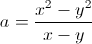If bothand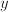are positive, what is the simplest form of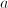?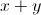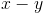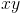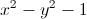Explanation: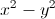can also be expressed as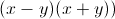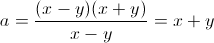Example Question #21 : Simplifying Expressions

Which of the following does not simplify to?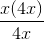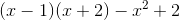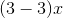All of these simplify toExplanation:

5x – (6x – 2x) = 5x – (4x) = x

(x – 1)(x + 2) - x2 + 2 = x2 + x – 2 – x2 + 2 = x

x(4x)/(4x) = x

(3 – 3)x = 0x = 0

Example Question #30 : Simplifying Expressions

Simplify the result of the following steps, to be completed in order:

2. Multiply the sum by 4

3. Add x to the product

4. Subtract x – y from the sum

28x + 13y

29x + 13y

28x + 12y

28x + 11y

28x – 13y

28x + 13y

Explanation:

Step 1: 7x + 3y

Step 2: 4 * (7x + 3y) = 28x + 12y

Step 3: 28x + 12y + x = 29x + 12y

Step 4: 29x + 12y – (x – y) = 29x + 12y – x + y = 28x + 13y